Examples

Chapter 6 Class 12 Application of Derivatives
Serial order wise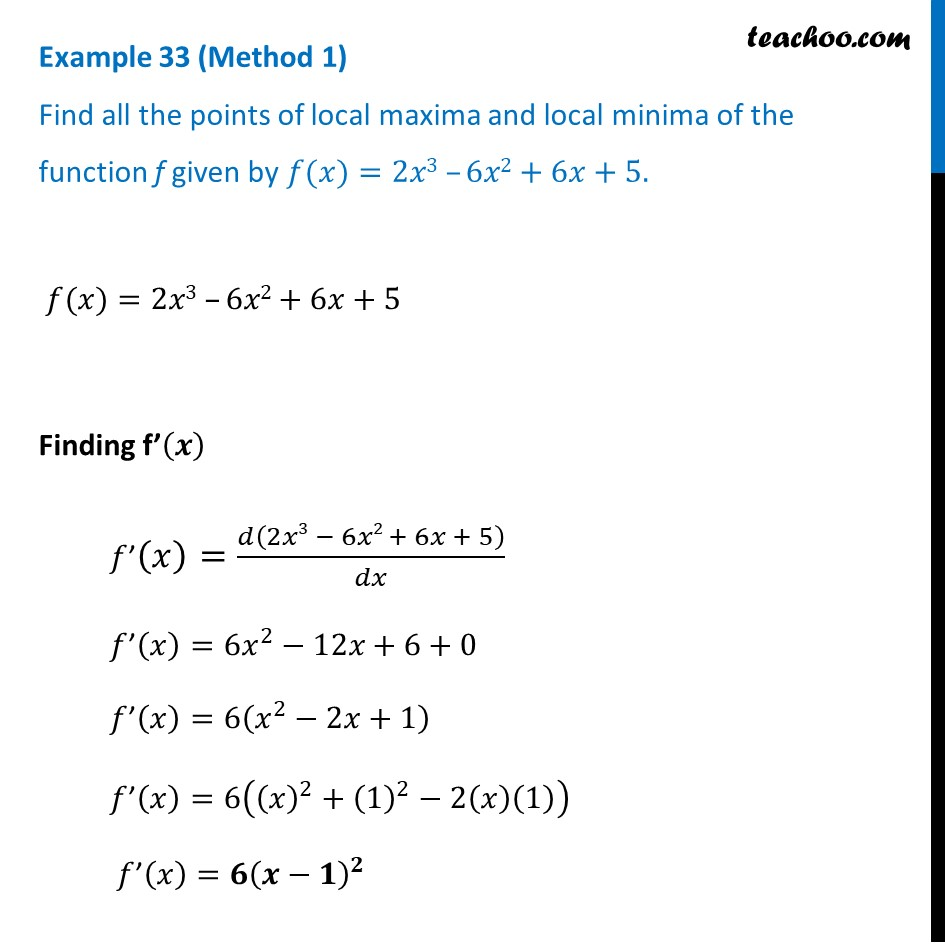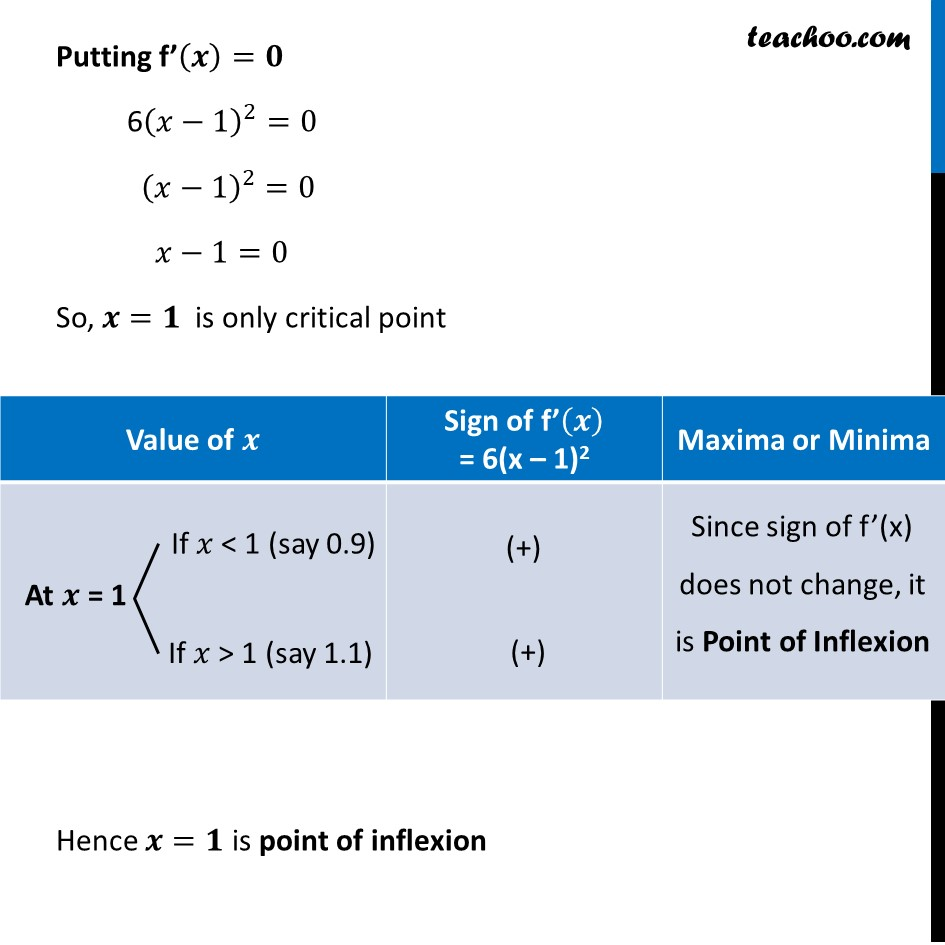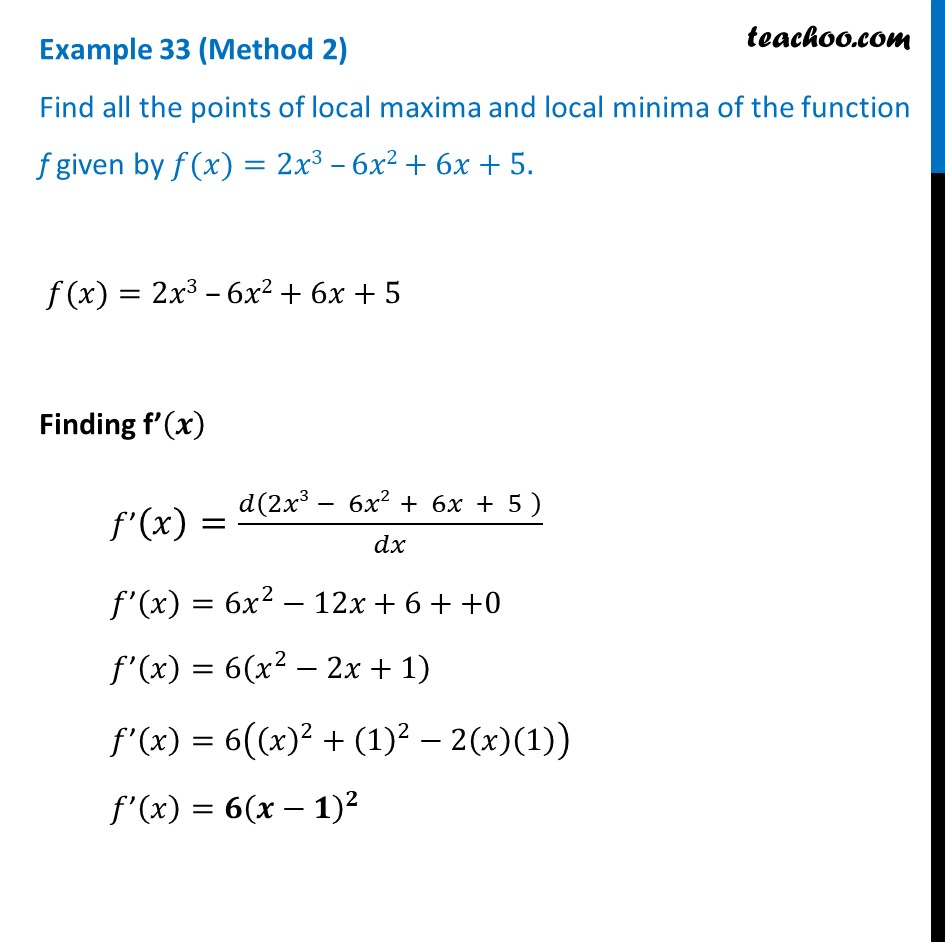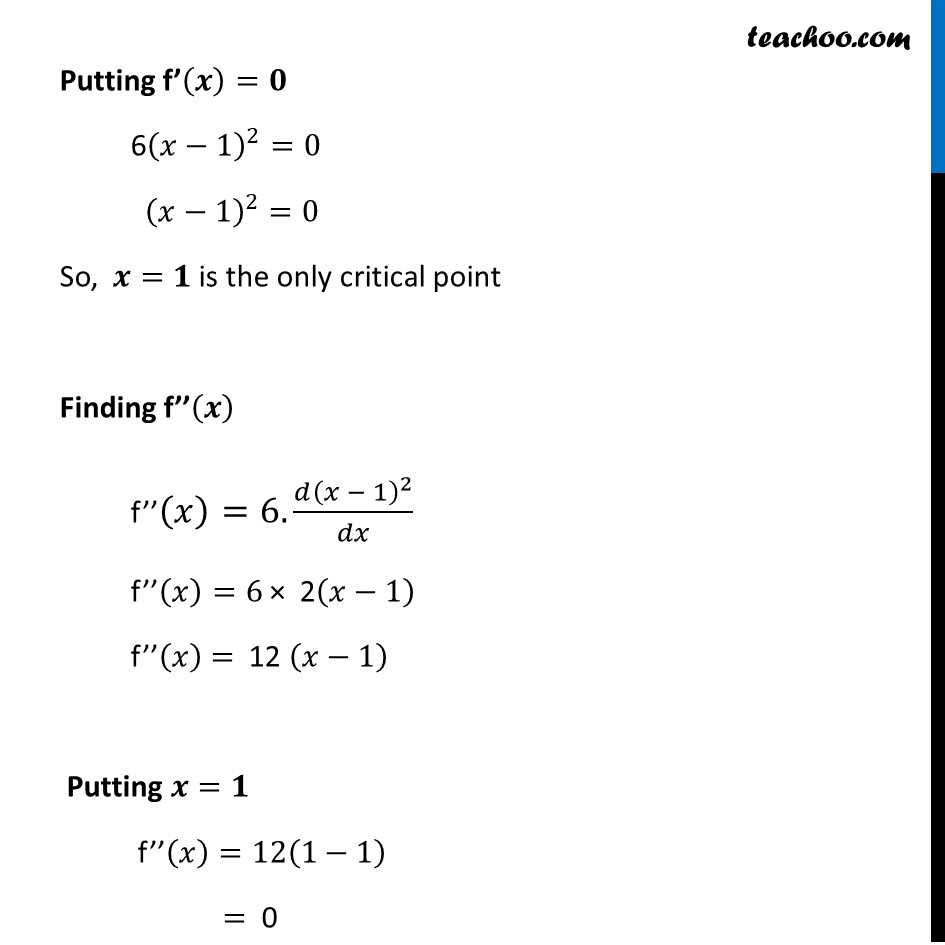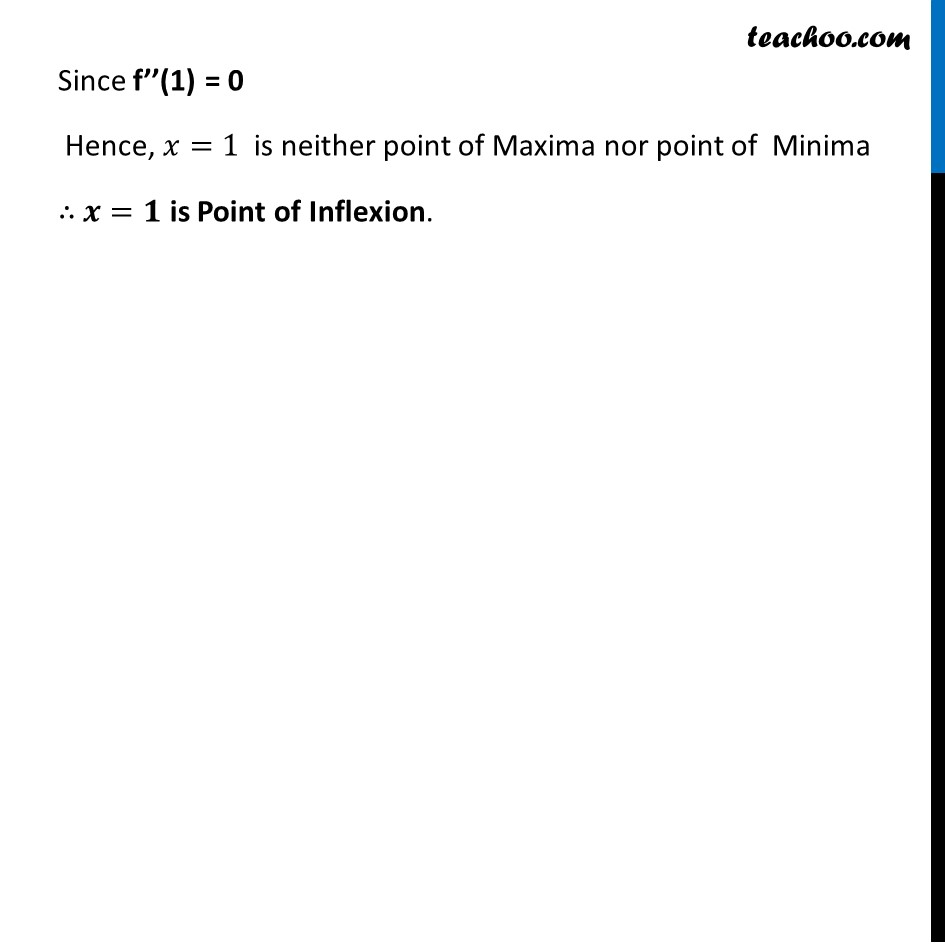Learn in your speed, with individual attention - Teachoo Maths 1-on-1 Class

### Transcript

Example 21 (Method 1) Find all the points of local maxima and local minima of the function f given by 𝑓(𝑥)=2𝑥3 –6𝑥2+6𝑥+5. 𝑓(𝑥)=2𝑥3 –6𝑥2+6𝑥+5 Finding f’(𝒙) 𝑓’(𝑥)=𝑑(2𝑥3 − 6𝑥2 + 6𝑥 + 5)/𝑑𝑥 𝑓’(𝑥)=6𝑥^2−12𝑥+6+0 𝑓’(𝑥)=6(𝑥^2−2𝑥+1) 𝑓’(𝑥)=6((𝑥)^2+(1)^2−2(𝑥)(1)) 𝑓’(𝑥)=𝟔(𝒙−𝟏)^𝟐 Putting f’(𝒙)=𝟎 6(𝑥−1)^2=0 (𝑥−1)^2=0 𝑥−1=0 So, 𝒙=𝟏 is only critical point Hence 𝒙=𝟏 is point of inflexion Example 21 (Method 2) Find all the points of local maxima and local minima of the function f given by 𝑓(𝑥)=2𝑥3 –6𝑥2+6𝑥+5. 𝑓(𝑥)=2𝑥3 –6𝑥2+6𝑥+5 Finding f’(𝒙) 𝑓’(𝑥)=𝑑(2𝑥3 − 6𝑥2 + 6𝑥 + 5" " )/𝑑𝑥 𝑓’(𝑥)=6𝑥^2−12𝑥+6++0 𝑓’(𝑥)=6(𝑥^2−2𝑥+1) 𝑓’(𝑥)=6((𝑥)^2+(1)^2−2(𝑥)(1)) 𝑓’(𝑥)=𝟔(𝒙−𝟏)^𝟐 Putting f’(𝒙)=𝟎 6(𝑥−1)^2=0 (𝑥−1)^2=0 So, 𝒙=𝟏 is the only critical point Finding f’’(𝒙) f’’(𝑥)=6.(𝑑(𝑥 − 1)^2)/𝑑𝑥 f’’(𝑥)=6 × 2(𝑥−1) f’’(𝑥) = 12 (𝑥−1) Putting 𝒙=𝟏 f’’(𝑥)=12(1−1) = 0 Since f’’(1) = 0 Hence, 𝑥=1 is neither point of Maxima nor point of Minima ∴ 𝒙=𝟏 is Point of Inflexion.